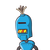# PA and PB are the tangents drawn to circle from external point P .PA = 6cm ,QR is another tangents touch the circle at S and inter

PA and PB are the tangents drawn to circle from external point P .PA = 6cm ,QR is another tangents touch the circle at S and intersect PA at Q and PB at R .The perimeter of triangle PQR is​

### 1 thought on “PA and PB are the tangents drawn to circle from external point P .PA = 6cm ,QR is another tangents touch the circle at S and inter”

1.Step-by-step explanation:

Correct option is

A

20

From the graph, we find some conclusion-

PA=PB=10cm

CE=CA

DE=DB

Perimeter of ΔPCD is,

=PC+PD+CD

⇒PC+PD+CE+ED

⇒PC+PD+CA+DB

⇒(PC+CA)(PD+DB)

⇒PA+PB

⇒10+10

⇒20 cm.